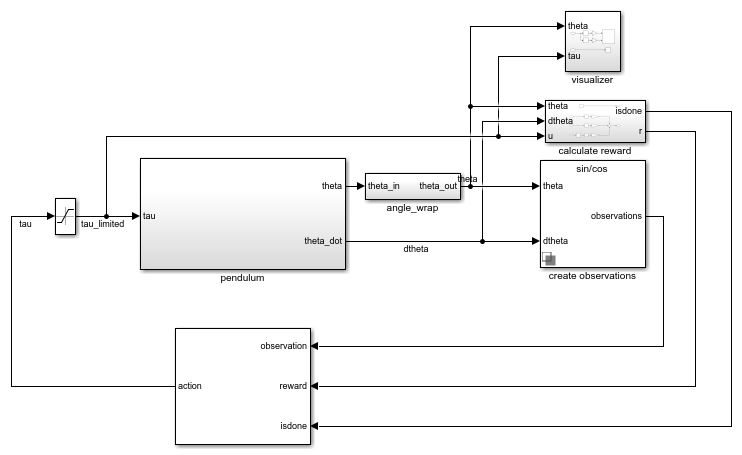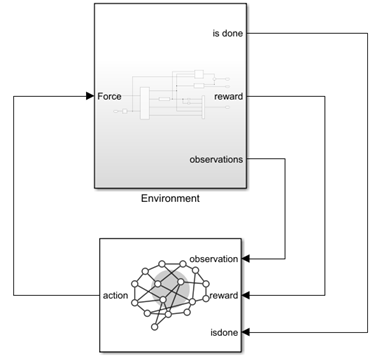Reinforcement Learning Toolbox™ software provides predefined Simulink® environments for which the actions, observations, rewards, and dynamics are already defined. You can use these environments to:

• Learn reinforcement learning concepts.

• Gain familiarity with Reinforcement Learning Toolbox software features.

• Test your own reinforcement learning agents.

You can load the following predefined Simulink environments using the `rlPredefinedEnv` function.

Simple pendulum Simulink modelSwing up and balance a simple pendulum using either a discrete or continuous action space.
Cart-pole Simscape™ modelBalance a pole on a moving cart by applying forces to the cart using either a discrete or continuous action space.

For predefined Simulink environments, the environment dynamics, observations, and reward signal are defined in a corresponding Simulink model. The `rlPredefinedEnv` function creates a `SimulinkEnvWithAgent` object that the `train` function uses to interact with the Simulink model.

This environment is a simple frictionless pendulum that initially hangs in a downward position. The training goal is to make the pendulum stand upright without falling over using minimal control effort. The model for this environment is defined in the `rlSimplePendulumModel` Simulink model.

`open_system('rlSimplePendulumModel')`There are two simple pendulum environment variants, which differ by the agent action space.

• Discrete — Agent can apply a torque of either Tmax, `0`, or -Tmax to the pendulum, where Tmax is the `max_tau` variable in the model workspace.

• Continuous — Agent can apply any torque within the range [-Tmax,Tmax].

To create a simple pendulum environment, use the `rlPredefinedEnv` function.

• Discrete action space

`env = rlPredefinedEnv('SimplePendulumModel-Discrete');`
• Continuous action space

`env = rlPredefinedEnv('SimplePendulumModel-Continuous');`

For examples that train agents in the simple pendulum environment, see:

#### Actions

In the simple pendulum environments, the agent interacts with the environment using a single action signal, the torque applied at the base of the pendulum. The environment contains a specification object for this action signal. For the environment with a:

For more information on obtaining action specifications from an environment, see `getActionInfo`.

#### Observations

In the simple pendulum environment, the agent receives the following three observation signals, which are constructed within the create observations subsystem.

• Sine of the pendulum angle

• Cosine of the pendulum angle

• Derivative of the pendulum angle

For each observation signal, the environment contains an `rlNumericSpec` observation specification. All the observations are continuous and unbounded.

For more information on obtaining observation specifications from an environment, see `getObservationInfo`.

#### Reward

The reward signal for this environment, which is constructed in the calculate reward subsystem, is

`${r}_{t}=-\left({\theta }_{t}^{2}+0.1\ast {\stackrel{˙}{\theta }}_{t}^{2}+0.001\ast {u}_{t-1}^{2}\right)$`

Here:

• θt is the pendulum angle of displacement from the upright position.

• ${\stackrel{˙}{\theta }}_{t}$ is the derivative of the pendulum angle.

• ut-1 is the control effort from the previous time step.

### Cart-Pole Simscape Model

The goal of the agent in the predefined cart-pole environments is to balance a pole on a moving cart by applying horizontal forces to the cart. The pole is considered successfully balanced if both of the following conditions are satisfied:

• The pole angle remains within a given threshold of the vertical position, where the vertical position is zero radians.

• The magnitude of the cart position remains below a given threshold.

The model for this environment is defined in the `rlCartPoleSimscapeModel` Simulink model. The dynamics of this model are defined using Simscape Multibody™.

`open_system('rlCartPoleSimscapeModel')`In the Environment subsystem, the model dynamics are defined using Simscape components and the reward and observation are constructed using Simulink blocks.

`open_system('rlCartPoleSimscapeModel/Environment')`There are two cart-pole environment variants, which differ by the agent action space.

• Discrete — Agent can apply a force of `15`, `0`, or `-15` to the cart.

• Continuous — Agent can apply any force within the range [`-15`,`15`].

To create a cart-pole environment, use the `rlPredefinedEnv` function.

• Discrete action space

`env = rlPredefinedEnv('CartPoleSimscapeModel-Discrete');`
• Continuous action space

`env = rlPredefinedEnv('CartPoleSimscapeModel-Continuous');`

For an example that trains an agent in this cart-pole environment, see Train DDPG Agent to Swing Up and Balance Cart-Pole System.

#### Actions

In the cart-pole environments, the agent interacts with the environment using a single action signal, the force applied to the cart. The environment contains a specification object for this action signal. For the environment with a:

For more information on obtaining action specifications from an environment, see `getActionInfo`.

#### Observations

In the cart-pole environment, the agent receives the following five observation signals.

• Sine of the pole angle

• Cosine of the pole angle

• Derivative of the pendulum angle

• Cart position

• Derivative of cart position

For each observation signal, the environment contains an `rlNumericSpec` observation specification. All the observations are continuous and unbounded.

For more information on obtaining observation specifications from an environment, see `getObservationInfo`.

#### Reward

The reward signal for this environment is the sum of three components (r = rqr + rn + rp):

• A quadratic regulator control reward, constructed in the ```Environment/qr reward``` subsystem.

`${r}_{qr}=-\left(0.1\ast {x}^{2}+0.5\ast {\theta }^{2}+0.005\ast {u}_{t-1}^{2}\right)$`

• An additional reward for when the pole is near the upright position, constructed in the `Environment/near upright reward` subsystem.

`${r}_{n}=10\ast \left(|\theta |<0.175\right)$`
• A cart limit penalty, constructed in the ```Environment/x limit penalty``` subsystem. This subsystem generates a negative reward when the magnitude of the cart position exceeds a given threshold.

`${r}_{p}=-100\ast \left(|x|\ge 3.5\right)$`

Here:

• x is the cart position.

• θ is the pole angle of displacement from the upright position.

• ut-1 is the control effort from the previous time step.